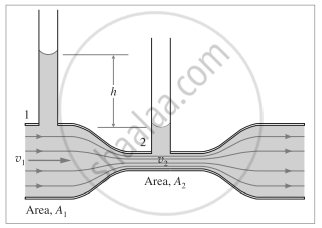Advertisement Remove all ads

# Why does the speed of a liquid increase and its pressure decrease when a liquid passes through constriction in a horizontal pipe? - Physics

Short Note

Why does the speed of a liquid increase and its pressure decrease when a liquid passes through a constriction in a horizontal pipe?

Advertisement Remove all ads

#### Solution

Consider a horizontal constricted tube.Let A1 and A2 be the cross-sectional areas at points 1 and 2, respectively. Let v1 and v2 be the corresponding flow speeds. ρ is the density of the fluid in the pipeline. By the equation of continuity,

v1A1 = v2A2        ....(1)

∴ "v"_2/"v"_1 = "A"_1/"A"_2 > 1   (∵ A1 > A2)

Therefore, the speed of the liquid increases as it passes through the constriction. Since the meter is assumed to be horizontal, from Bernoulli’s equation we get,

"p"_1 = 1/2rho"v"_1^2 = "p"_2 + 1/2rho_2^2

∴ "p"_1 + 1/2rho"v"_1^2 = "p"_2 + 1/2rho"v"_1^2 ("A"_1/"A"_2)^2  .....[from eq.(1)]

∴ "p"_1 - "p"_2 = 1/2"pv"_1^2 [("A"_1/"A"_2)^2 - 1]    ...(2)

Again, since A1 > A2, the bracketed term is positive so that p1 > p2 . Thus, as the fluid passes through the constriction or throat, the higher speed results in lower pressure at the throat.

Concept: Critical Velocity and Reynolds Number
Is there an error in this question or solution?
Advertisement Remove all ads

#### APPEARS IN

Balbharati Physics 12th Standard HSC Maharashtra State Board
Chapter 2 Mechanical Properties of Fluids
Exercises | Q 5 | Page 54
Advertisement Remove all ads
Advertisement Remove all ads
Share
Notifications

View all notifications

Forgot password?